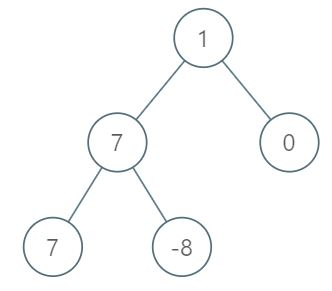1161. 最大层内元素和

# 1161. 最大层内元素和#

• 标签：树、深度优先搜索、广度优先搜索、二叉树
• 难度：中等

## 题目大意 #

• 树中的节点数在 $[1, 10^4]$ 范围内。
• $-10^5 \le Node.val \le 10^5$。

• 示例 1：1 2 3 4 5 6 7  输入：root = [1,7,0,7,-8,null,null] 输出：2 解释： 第 1 层各元素之和为 1， 第 2 层各元素之和为 7 + 0 = 7， 第 3 层各元素之和为 7 + -8 = -1， 所以我们返回第 2 层的层号，它的层内元素之和最大。 
• 示例 2：
 1 2  输入：root = [989,null,10250,98693,-89388,null,null,null,-32127] 输出：2 

## 解题思路 #

### 思路 1：二叉树的层序遍历 #

1. 利用广度优先搜索，在二叉树的层序遍历的基础上，统计每一层节点和，并存入数组 $levels$ 中。
2. 遍历 $levels$ 数组，从 $levels$ 数组中找到最大层和 $max\underline{}sum$。
3. 再次遍历 $levels$ 数组，找出等于最大层和 $max\underline{}sum$ 的那一层，并返回该层序号。

### 思路 1：代码 #

  1 2 3 4 5 6 7 8 9 10 11 12 13 14 15 16 17 18 19 20 21 22 23 24 25 26 27 28 29 30 31  # Definition for a binary tree node. # class TreeNode: # def __init__(self, val=0, left=None, right=None): # self.val = val # self.left = left # self.right = right class Solution: def levelOrder(self, root: TreeNode) -> List[List[int]]: if not root: return [] queue = [root] levels = [] while queue: level = 0 size = len(queue) for _ in range(size): curr = queue.pop(0) level += curr.val if curr.left: queue.append(curr.left) if curr.right: queue.append(curr.right) levels.append(level) return levels def maxLevelSum(self, root: Optional[TreeNode]) -> int: levels = self.levelOrder(root) max_sum = max(levels) for i in range(len(levels)): if levels[i] == max_sum: return i + 1 

### 思路 1：复杂度分析 #

• 时间复杂度：$O(n)$。其中 $n$ 是二叉树的节点数目。
• 空间复杂度：$O(n)$。